Next: Effect of sources evolution Up: Evolution of the Double Previous: Evolution of the Double

## Calculation of Binary Neutron Star Coalescence Rates

It is reasonable to expect a priori that the evolution of events is strongly time dependent, so the star formation history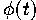in a galaxy is another important parameter. Therefore, by calculating the evolution of events after a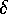-function like star formation burst,Ā one gets a Green functionfor any arbitrary star formation history.

At the one extreme we assume instantaneous star formation at a particular redshift resulting in a stellar system which we call ``elliptical''Ā since stars in elliptical galaxies to a good approximation can be assumed to be old and little if any additional star formation is occuring today. Thus the event rates in ``ellipticals'' can be described by the computed Green function. At the other extreme, constant star formation (= const) would result in ``spiral''-like stellar systems.Ā Irregular galaxies have probably also an irregular star formation history, but their contribution to the overall event rate hardly exceeds their fraction among all galaxies, that is 5-7 percent, so we neglect them in this context.

The source evolution in a galaxy with given star formation rateis thus calculated as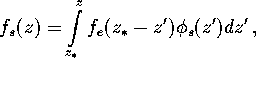whereis the redshift at the turn-on of star formation, which is the second important parameter of the model. Assuming to the zero approximation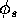Ā= const, we getWe parametrize the star formation history in the Universe by the fractional part of the luminous baryonic matter entering into elliptical galaxies,Ā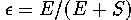, where E and S refer to ``elliptical'' galaxies (i.e. without additional star formation) and ``spiral'' galaxiesĀ (with a constant star formation rate). In fact, this parameter must be higher than the presently observed fraction of elliptical galaxies, as any galaxy must have had more violent star formation at earlier time. The galaxies are supposed to be formed at the momentwith the initial star formation during first 500ĀMyr. The mean number of galaxies each of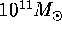was takenĀMpc(this roughly corresponds to a density of baryonic matter in galaxies without hidden mass of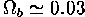).

We make usual assumptions about initial distributions of binary stars (see SectionĀ6) similar to those presently observed in our Galaxy (a Salpeter function for massof the primary component,Ā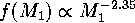and a flat distribution of the initial binary separations A:). Based on findings by the Scenario Machine analysis of the evolutionary scenario by Lipunov et al. (1995b) (Section 7), we take the initial mass ratio distribution in a power-law formand the efficiency coefficient of angular momentum removal at the common envelopeĀ stage(as defined by van den Heuvel 1994).Next: Effect of sources evolution Up: Evolution of the Double Previous: Evolution of the Double

Mike E. Prokhorov
Sat Feb 22 18:38:13 MSK 1997